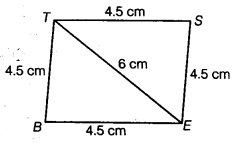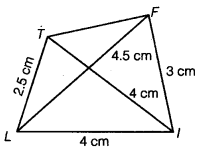# Class 8 Maths NCERT Solutions for Chapter – 4 Practical Geometry Ex – 4.1

## Practical Geometry

Question 1.

AB = 4.5 cm
BC = 5.5 cm
CD = 4 cm
AC = 7 cm

JU = 3.5 cm
UM = 4 cm
MP = 5 cm
PJ = 4.5 cm
PU 6.5 cm

(iii) Parallelogram MORE
OR = 6 cm
RE = 4.5 cm
EO = 7.5 cm

(iv) Rhombus BEST
BE = 4.5 cm
ET = 6 cm

Solution.

(i)
First we draw a rough sketch of a quadrilateral ABCD and write down its dimensions as shown.
We may divide it into two conveniently constructible As ABC and ACD.

Steps of Construction :

• Draw AC = 7 cm.
• With A as centre and radius 4.5 cm, draw an arc (below AC).
• With C as centre and radius 5.5 cm, draw another arc cutting the previous arc at B.
• Join AB and BC.
• With A as centre and radius 6 cm, draw an arc (above AC).
• With C as centre and radius 4 cm, draw another arc cutting the previous arc at D.
Then, ABCD is the required quadrilateral.

(ii) First we draw a rough sketch of a quadrilateral JUMP and write down its dimensions as shown.
We may divide it into two conveniently constructible As PJU and PMU.

Steps of Construction :• Draw PU = 6.5 cm.
• With P as centre and radius 4.5 cm, draw an arc (below PU).
• With U as centre and radius 3.5 cm, draw another arc cutting the previous arc at J.
• Join PJ and JU.
• With P as centre and radius 5 cm, draw an arc (above PU).
• With U as centre and radius 4 cm, draw another me cutting the previous arc at M.
• Join PM and UM.
Then, JUMP is the required quadrilateral.

(iii) Draw a rough sketch of the’ required parallelogram and write down the given dimensions.

Steps of Construction :• Draw OR = 6 cm.
• With O as centre and radius 7.5 cm, draw an arc.
• With R as centre and radius 4.5 cm, draw another arc to cut the previous arc at E.
• Join OE and RE.
• With 0 as centre and radius 4 5 cm 4.5 cm, draw an arc.
• With E as centre and radius 6 cm, draw another arc to cut the previous arc at M.
• Join MO and ME.
Then, MORE is the required parallelogram.

(iv) Draw a rough sketch of the required rhombus and write down the given dimensions.

Steps of Construction :• Draw BE = 4.5 cm.
• With B as centre and radius 4.5 cm, draw an arc. 4 5 cm
• With E as centre and radius 6 cm, draw another arc to cut the previous arc at T.
• Join ST and ET.
• With T as centre and radius 4.5 cm, draw an arc.
• With E as centre and radius 4.5 cm, draw another arc to cut the previous arc at S.
• Join TS and ES.
Then, BEST is the required rhombus.